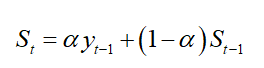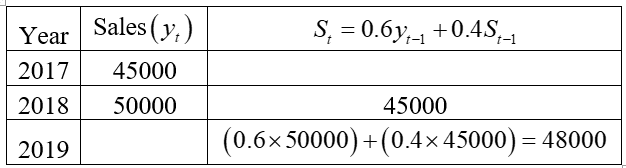# Simple Moving Average Sales forecast in 4-1 equal \$65,500. Show by hand 4-2 Using exponential smoothing with a weight of 0.6 on actual values: (a) If sales are \$45,000 and \$50,000 for 2017 and 2018, what would you forecast for 2019 ? (The first forecast is equal to the value of the preceding year.)  (b) Given this forecast and actual 2019 sales of \$53,000, what would you then forecast for 2020?   4-3 In Problem 4-1, taking actual 2015 sales of \$48,000bas the forecast for 2016, what sales would you for cast for 2017, 2018, and 2019, using exponential smoothing and a weight on actual values of (a) 0.4 and (b) 0.8 ?

Question
1 views

Simple Moving Average Sales forecast in 4-1 equal \$65,500. Show by hand 4-2 Using exponential smoothing with a weight of 0.6 on actual values: (a) If sales are \$45,000 and \$50,000 for 2017 and 2018, what would you forecast for 2019 ? (The first forecast is equal to the value of the preceding year.)  (b) Given this forecast and actual 2019 sales of \$53,000, what would you then forecast for 2020?   4-3 In Problem 4-1, taking actual 2015 sales of \$48,000bas the forecast for 2016, what sales would you for cast for 2017, 2018, and 2019, using exponential smoothing and a weight on actual values of (a) 0.4 and (b) 0.8 ?

check_circle

Step 1

Hello! As you have posted 2 different questions, we are answering the first question (4-2). In case you require the unanswered question also, kindly re-post them as separate question.

Step 2

4-2)

(a)

Given that,

The sales for 2017 is \$45,000.

The sales for 2018 is \$50,000.

Exponential smoothing weight as 0.6. That is, α=0.6.

The formula for Exponential smoothing:Thus, the forecast sales for 2019 is \$48,000.

...

### Want to see the full answer?

See Solution

#### Want to see this answer and more?

Solutions are written by subject experts who are available 24/7. Questions are typically answered within 1 hour.*

See Solution
*Response times may vary by subject and question.
Tagged in